Thank you for visiting nature.com. You are using a browser version with limited support for CSS. To obtain the best experience, we recommend you use a more up to date browser (or turn off compatibility mode in Internet Explorer). In the meantime, to ensure continued support, we are displaying the site without styles and JavaScript.

# Hyperthermic effects of dissipative structures of magnetic nanoparticles in large alternating magnetic fields

## Abstract

Targeted hyperthermia treatment using magnetic nanoparticles is a promising cancer therapy. However, the mechanisms of heat dissipation in the large alternating magnetic field used during such treatment have not been clarified. In this study, we numerically compared the magnetic loss in rotatable nanoparticles in aqueous media with that of non-rotatable nanoparticles anchored to localised structures. In the former, the relaxation loss in superparamagnetic nanoparticles has a secondary maximum because of slow rotation of the magnetic easy axis of each nanoparticle in the large field in addition to the known primary maximum caused by rapid Néel relaxation. Irradiation of rotatable ferromagnetic nanoparticles with a high-frequency axial field generates structures oriented in a longitudinal or planar direction irrespective of the free energy. Consequently, these dissipative structures significantly affect the conditions for maximum hysteresis loss. These findings shed new light on the design of targeted magnetic hyperthermia treatments.

## Introduction

Tumour-targeted magnetic hyperthermia has recently attracted much attention1. Preferential accumulation of magnetic nanoparticles in tumour tissue is achieved by conjugating nanoparticles with tumour-homing peptides2 or antibodies3. When the accumulated nanoparticles are exposed to a large alternating magnetic field, H = Hacsin(2πf·t), where Hac is the amplitude of the field, f is the frequency and t is time, they begin to rotate because of magnetic torque. Simultaneously, the direction of the magnetic moment, μ , in each nanoparticle reverses with a certain probability. Consequently, heat equivalent to the magnetic loss dissipates locally in the tumour tissue. If the properties of the irradiated field are limited (i.e., Hac·f < constant)1 to ensure biomedical safety, then nanoparticles that maximise the in vivo efficiency of heat dissipation, PH/(Hac·f), are required, where PH is the specific energy dissipation rate (specific loss power) per unit mass of nanoparticles. The actual rotations of the nanoparticles are disordered because the microviscosity of the local environment in cancer cells is not constant4,5 and effective elasticity depends on the binding conditions between nanoparticles and membranes. To minimise the effect of irregular rotations in magnetic hyperthermia, two guiding principles have been proposed on the basis of simple models that consider a linear response of thermodynamic equilibrium states or magnetic field-driven reversals.

The first guiding principle6 is to use the relaxation loss in superparamagnetic iron oxide nanoparticles (SPIONs) with a sufficiently low energy barrier ΔU for reversal. If a linear response of their thermodynamic equilibrium state is considered at low Hac, the out-of-phase component of AC susceptibility χ″ can be expressed as follows: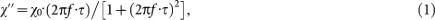where χ 0 is the initial susceptibility per unit mass of SPIONs. When reversal and rotation occur in a nanoparticle in parallel, the characteristic time τ is given by the following equation: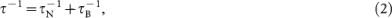where τ N is the Néel relaxation time for reversal and τ B is the Brownian relaxation time for rotation. Consequently, the heating efficiency PH/(Hac·f ) = πμ0 χ″·Hac for individual monodisperse nanoparticles has a single maximum at the peak frequency 2πfp = τ −1. For a sufficiently small SPION, τ is determined only by τ N because τ N is much shorter than τ B. In this case, it has been assumed that the conditions for maximising the efficiency are unaffected by uncontrolled rotation of the nanoparticles.

However, in some experiments, dual peaks have been observed for the frequency dependence of χ″ PH/(Hac·f )7,8 despite the prediction of a single peak at a 2πfp value of τ −1 ( = τ N−1 + τ B−1). For this reason, size distribution7 or aggregation8 of the nanoparticles was considered based on the linear response theory. In an earlier study7, the low-frequency peak observed for the susceptibility was attributed to Brownian relaxation of larger nanoparticles, while the high-frequency peak was attributed to Néel relaxation of smaller nanoparticles. In another study8, the low- and high-frequency peaks were attributed to individual and agglomerated nanoparticles, respectively. Thus the observed dual peaks have been theoretically explained by the coexistence of two kinds of nanoparticles. In other words, these explanations are based on the assumption that a single kind of nanoparticle will produce only a single peak at τ −1 ( = τ N−1 + τ B−1). However, this assumption has never been theoretically verified under a large AC magnetic field, where the linear response theory does not hold.

The second guiding principle is to use hysteresis loss in ferromagnetic nanoparticles9. In mechanical models such as the Stoner–Wohlfarth model for single domain particles, μ is reversed in the time scale of Larmor precession (picoseconds) when ΔU disappears at the switching field Hsw, because thermal fluctuations are not considered. Such fast reversals are considered to dominate the response to high frequency AC magnetic field because the Brownian relaxations of large ferromagnetic nanoparticles are generally slow compared with the oscillation of the field. In this case, the work done in one cycle is given by the area inside the hysteresis loop, ζ·Ms·Hsw, where Ms is the spontaneous magnetisation and ζ is a coefficient related to the rectangularity of the loop. In the simple case of rectangular hysteresis loops, ζ is 0 for Hac < Hsw and 4 for HacHsw. Consequently, the maximum efficiency, PH/(Hac·f ) = ζ·Ms·(Hsw/Hac)/ρ, where ρ is the density of magnetite, is achieved when Hac is adjusted to Hsw. Because Hsw depends on the magnetic anisotropy field HK specific to each nanoparticle, it has been assumed that, in cases where reversal is much faster than rotation, the amount of hysteresis loss is unaffected by the inhomogeneous rotations of nanoparticles in cancer cells.

In recent experimental studies10,11, the observed PH of immobilised ferromagnetic nanoparticles was lower than that of the same nanoparticles dispersed in a fluid. Kim et al.10 attributed the difference to variation in the rates of convective heat transfer. Müller et al.11 suggested that the orientation or agglomeration of the nanoparticles, or interaction effects, may be responsible for the observed difference. The orientation of nanoparticles is important because it is related to the magnetic torque intrinsic in rotatable ferromagnetic nanoparticles. However, there has been no theoretical study on magnetic field-driven reversals of μ in ferromagnetic nanoparticles with easy axes that simultaneously rotate under the magnetic torque.

There are many reported inconsistencies between experimental results and predictions based on the above two guiding principles for optimising hyperthermia treatment. These guiding principles are based on the simple models established at the two limits: in zero magnetic field or at zero temperature. Under the conditions for hyperthermia (Hac ≠ 0, T ≠ 0), where T is temperature, the validity of the guiding principles has not been theoretically verified even for an ideal system of non-interacting monodisperse nanoparticles. Consequently, we attempted to simulate the thermally assisted magnetic response of individual superparamagnetic/ferromagnetic iron oxide nanoparticles exposed to a large AC magnetic field like that used in hyperthermia treatment. The simulation was performed in the following two extreme cases: non-rotatable nanoparticles strongly anchored to structures resembling organelles and rotatable nanoparticles in an aqueous phase mimicking cytoplasm. In the simulations, the thermally activated reversals of μ were calculated between the meta-stable directions. Simultaneously, the rotations of the spheroidal nanoparticles were computed in the inertialess limit (Brownian dynamics simulation), where the frictional torque always balances with magnetic torque and with Brownian torque (details are reported in the Methods section). The results allow examination of whether the relaxation loss for τ N « τ B and the hysteresis loss at HacHsw are independent of the ability of the nanoparticles to rotate under the conditions for hyperthermia treatment.

## Results

The magnetic response to an AC magnetic field Hacsin(2πf·t) at T = 310 K was simulated for individual monodisperse spheroidal magnetite nanoparticles with non-magnetic surfactant layers in non-rotatable and rotatable situations (see the Methods section for details). Results are presented for the following representative nanoparticles: nearly spherical nanoparticles with an aspect ratio, κ , of 1.1 and an equatorial diameter, 2RM, of 18 nm and elongated spheroidal particles with κ = 1.4 and 2RM = 24 nm. The parameters of these nanoparticles are summarised in Table 1. The former nanoparticles with τ N (Hac = 0) of 20 ns can be considered as typical SPIONs, while the latter with τ N (Hac = 0) of 2×107 s (1 year) can be regarded as typical ferromagnetic nanoparticles in the frequency range of hyperthermia treatment ((2πf )−1 of approximately 1 μs). Results for nanoparticles with other sizes and shapes are shown in the Supplementary Information.

The magnetisation curves of the non-rotatable nearly spherical nanoparticles at low Hac (1 kA/m) are shown in Fig. 1. A linear response without hysteresis was observed at f = 100 kHz. Such superparamagnetic behaviour is reasonable because the estimated τ N(Hac = 0) for the nanoparticles is 20 ns. Hysteresis appeared in the curves at f = 1,000 kHz. As f increased further, the area inside the hysteresis loop grew. This area corresponds to the work done in one cycle. Therefore, PH/(Hac·f ) also increased with f and a single maximum was observed at a peak frequency, fp, of 10,000 kHz (Fig. 1 (b)). Figure 2(a) shows the Hac dependence using a contour plot of PH/(Hac·f ). As Hac increased, fp shifted towards higher frequencies. As indicated by the dashed line in Fig. 2(a), this shift can be explained by the values of τ N(Hac) calculated using the conventional Brown's equation as follows12: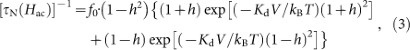where h is μH/(2 KdV), Kd is the shape anisotropy constant, V is the volume of the magnetic core and kB is the Boltzmann constant. Therefore, the emergence of a single peak in PH/(Hac·f ) can be attributed to Néel relaxation loss, as expected for SPIONs.

For the nearly spherical nanoparticles with a low Hac (1 kA/m), the magnetisation curves in the rotatable case were the same as those in the non-rotatable case (see Fig. 1). An equivalent maximum appeared in the f-dependence of PH/(Hac·f ) in the linear response range (Hac = 1 kA/m) (Fig. 1(b)). This behaviour is consistent with the above assumption because the estimated τ N (Hac = 0) of 20 ns is much shorter than τ B = 8 μs (Table 1). The shift of this peak with increasing Hac is analogous to that in the non-rotatable case (Fig. 2(b)). However, another maximum of PH/(Hac·f ) was observed at Hac = 8−16 kA/m and f = 30 kHz in the contour plot shown in Fig. 2(b). A secondary maximum like this has not previously been theoretically predicted for individual monodisperse nanoparticles. Figure 3(a) shows the magnetisation curves calculated under these conditions. Unlike the non-rotatable case, an S-shaped hysteresis loop without remanence existed. At the same time, the mean orientation of the long (easy) axes of the nanoparticles showed butterfly-shaped hysteresis, as shown Fig. 3(b). Because such behaviour cannot be explained using the present linear response theory, its origin is discussed in the next section from the viewpoint of the rotation of the long axis of SPION.

The magnetisation curves at f = 10,000 kHz for the elongated spheroidal nanoparticles, which are typical ferromagnetic nanoparticles, in the non-rotatable case are shown in Fig. 4(a). The curve was reversible at Hac = 20 kA/m and hysteresis appeared in the curve at Hac = 26 kA/m. As Hac increased further, the area inside the hysteresis loop grew. When Hac became larger than 32 kA/m, the expansion of the area was saturated and the shape of the magnetisation curve approached that predicted by the Stoner–Wohlfarth model. PH/(Hac·f ) was almost zero at low Hac, then at approximately 30 kA/m it began increasing rapidly with Hac, followed by a gradual decreases with increases in Hac (Fig. 4(b)). This behaviour depends weakly on the frequency (Fig. 5(a)). In mechanical models that do not consider thermal fluctuation, a hysteresis loop appeared when Hac was higher than Hsw. Because Hsw of ferromagnetic nanoparticles with randomly oriented easy axes ranges from HK/2 = 29 kA/m to HK = 57 kA/m and is often close to 0.5 HK, that PH/(Hac·f ) is almost independent of frequency at Hac > 30 kA/m in the non-rotatable ferromagnetic nanoparticles is consistent with the properties expected for the hysteresis loss.

Figure 6 shows the magnetisation curves for rotatable elongated spheroidal nanoparticles at f = 10,000 kHz. Because the magnetic response slowly changed after the AC magnetic field was applied at t = 0, transient variations of the hysteresis loops are observed. The shape of the major hysteresis loop at Hac = 60 kA/m was initially consistent with that predicted by the Stoner–Wohlfarth model with randomly oriented easy axes. However, the remanence of the major loop gradually increased from 0.5 Ms to Ms. In other words, the major loop became squarer and the area inside the loop increased with time. In comparison, the remanence of the minor loop at Hac = 26 kA/m gradually decreased and the area became smaller over time. As shown in Fig. 6(c) and (d), the long (easy) axes of the nanoparticles gradually turned when the variations of the loops proceeded (see the next section for details). Consequently, the increased area of the major hysteresis loops and decreased area of the minor loops caused the maximum of PH/(Hac·f ) to shift towards higher Hac compared with the non-rotatable case (see arrows in Fig. 4(b)). Note that reversals occurred every hundred nanoseconds (1/f ), while rotations took several microseconds (Fig. 6(c)). Thus, the assumption that the amount of hysteresis loss is unaffected by the rotation of nanoparticles when reversal is significantly faster is invalid for ferromagnetic nanoparticles in large AC magnetic fields at high frequencies.

Before discussing this novel phenomenon observed at high frequencies, the other important variation in the contour plot of PH/(Hac·f ) (Fig. 5) that occurred because of the ability of the ferromagnetic nanoparticles to rotate at lower frequencies shall be examined. The maximum of PH/(Hac·f ) shifted toward lower Hac below 100 kHz for the rotatable elongated spheroidal nanoparticles, while it stayed between HK/2 and HK in the non-rotatable case. Figure 7(a) shows the magnetisation curve obtained when Hac = 16 kA/m and f = 30 kHz. The curve in the rotatable case had an obvious hysteresis loop with a large remanence in the steady state, but there was no hysteresis observed for the non-rotatable situation. Because Hac = 16 kA/m is much smaller than HK/2, no reversals of μ occur at any orientation of the easy axis so hysteresis is not observed for the latter case. Figure 7(b) shows the variation of <cosθ> in the rotatable case, where <cosθ> (0 < θ < π/2) is the mean angle between the magnetic field and the long axes of the spheroidal nanoparticles. Note that <cosθ> is synchronised with |M/Ms| = |cosψ|, where ψ is the angle between μ and H . This fact indicates that the hysteresis in the rotatable case (Fig 7(a)) is mainly caused by the rotation of the easy axis where the direction of μ is fixed. Consequently, heat equivalent to the hysteresis loss dissipates even at Hac < HK/2.

For Brownian relaxation, τ B can be expressed as follows: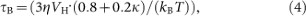where η is the viscosity of the surrounding medium and VH is the hydrodynamic volume. In equation (4), the frictional torque for spheroids, described in the Methods section, is considered. For the elongated spheroidal nanoparticle, (2πτ B)−1 is calculated to be 8 kHz. This value is too low to cause the nanoparticle to rotate at 30 kHz. Therefore, Yoshida et al.13 also took into account the rotation caused by magnetic torque, μ (t) × Η (t). They concluded that the area of the hysteresis loop was maximised as follows: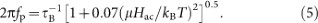The location of the peak in PH/(Hac·f ) below 100 kHz can be explained by this equation, as shown in Fig. 5(b). An expression that describes all of the variation in the position of the primary peak of PH/(Hac·f ) is desirable and equation (5) can be rewritten as follows: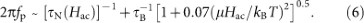This equation is an extended relationship of τ −1 = τ N−1 + τ B−1 for a large AC magnetic field. This expression is for the primary maximum; the secondary maximum is discussed later. The second term of equation (6) can be approximated to 0.1μHac/(ηVH) for μHac/kBT » (0.07)−0.5 and κ 1. On the other hand, τ N(Hac) of ferromagnetic nanoparticles becomes extremely short only when the energy barrier disappears between HK/2 and HK. Therefore, the changeover between the two terms in the equation (6) generally occurs at HacHK/2 and 2πf ≈ 0.1μHK/(2ηVH) = 0.1 KdV/(ηVH). For the elongated spheroidal particles (κ = 1.4, Kd = 16 kJ/m3 and V/VH = 0.3) in a liquid phase with η = 1 mPa·s, the values of Hac and f are 29 kA/m and 76 kHz. Such a changeover around 100 kHz occurs for ferromagnetic nanoparticles of any size, as long as the conditions, Kd, V/VH and η, are constant. We must keep in mind that, even when ferromagnetic nanoparticles are large enough for their Brownian relaxation to be negligible, the magnetic torque caused by the large AC magnetic field can easily rotate such nanoparticles in the liquid phase at a time scale of microseconds. This knowledge is helpful when considering the frequency for hyperthermia treatment, even if it is obtained for a simplified system.

In summary, most of the simulated results, including significant variations for ferromagnetic nanoparticles exposed to a low-frequency AC magnetic field, can be explained using the existing models. The two essential exceptions are as follows:

1. a

a secondary maximum in the relaxation loss for SPIONs exposed to a low-frequency AC magnetic field and

2. b

a shift of the maximum hysteresis loss caused by the ability of typical ferromagnetic nanoparticles exposed to a high-frequency AC magnetic field to rotate.

These novel phenomena are discussed in detail in the following section.

## Discussion

The two novel phenomena of rotatable nanoparticles in a large AC magnetic field described above cannot be explained by simple models that consider a linear response of thermodynamic equilibrium states or magnetic field-driven reversals. In this section, we shall further discuss these atypical responses from the viewpoint of the orientation of the long (easy) axis. First, we begin with the appearance of a secondary maximum of PH/(Hac·f ) near Hac = 8 kA/m and f = 30 kHz for the nearly spherical nanoparticles (typical SPIONs) where an S-shaped hysteresis loop without remanence was obtained (Fig. 3). We must recall that the variation in <cosθ> showed butterfly-shaped hysteresis under these conditions. This behaviour explains the atypical magnetic response in the period f−1 (33 μs) (Fig. 3(b)). Initially (at t = 0), no magnetisation exists because the occupation probabilities of μ in the two stable directions parallel to the long (easy) axis are equalised in a zero magnetic field. As H increases, the occupation probability in the more stabilised direction immediately increases because of reversals on a time scale of τ N (≤ 20 ns). The reversed μ in the stabilised direction is not completely parallel to H , ψ ≠ 0 and the magnetic torque μHsinψ turns the long (easy) axis towards the direction of the field. If we neglect Brownian torque λ (t) (see equation (11) in the Methods section), the angular velocity of the rotation due to magnetic torque can be expressed as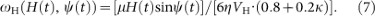Hence, ω H(H(t), ψ(t)) increases in proportion to the field amplitude H = Hacsin(2πf·t). For example, ω H(H, ψ = π/4) is 0.15×106 rad/s when H (t = 1/4f ≈ 8 μs) is 8 kA/m. Therefore, rotation is not negligible in the peak period of the oscillations of H. Subsequently, H decreases to zero at t = 1/2f ≈ 17 μs and the occupation probabilities are again equalised because reversal is rapid, so the magnetic torque disappears. Alternatively, the Brownian torque randomises the orientation of the long axis on a time scale of τ B ( = 8 μs). Therefore, competition between the magnetic and Brownian torques can cause the butterfly-shaped hysteresis of <cosθ>. Because the equilibrium magnetisation of SPIONs with easy axes parallel to H is higher than that of randomly oriented SPIONs14, the magnetisation curve shows hysteresis without remanence. Consequently, a secondary maximum appears for the rotatable SPIONs even though τ N « τ B.

Next, we investigate the influence of the ability of elongated spheroidal nanoparticles to rotate under a high-frequency AC magnetic field on the shift of the maximum PH/(Hac·f ). As shown in Fig. 6(a) and (b), the magnetisation curves varied after an AC magnetic field was applied at t = 0. At the same time, transient variations also occurred in <cosθ>, as shown in Fig. 6(c). In the case of the major loop at Hac = 60 kA/m, <cosθ> gradually increased from 0.5 to 0.95. In other words, the long (easy) axis became oriented towards the direction parallel to H . The characteristic time, τ rot, was estimated to be 1.3 μs using the approximation of exponential decay. Note that the direction of μ is not completely parallel to H even for HHK, even though μ is already reversed for all of the nanoparticles. Because sin ψ is 0.43 when cos ψ is 0.9, a large magnetic torque can turn the long axis even if the magnetisation is almost saturated after reversals at H HK. Indeed, τ rot at HacHK is comparable to the reciprocal of typical values of ω H(H = Hac, sin ψ = 0.43) (Fig. 6(d)). Therefore, these transient variations can be attributed to the longitudinal orientation being adopted preferentially because of the magnetic torque.

In the minor loops at Hac = 26 kA/m, the remanence of the rotatable nanoparticles decreased gradually with time (Fig. 6(b)) and <cosθ> simultaneously decreased from 0.5 (Fig. 6(c)). The long axis was oriented perpendicular to H during this period, although the longitudinal orientation is preferred when the Zeeman energy is considered. It should be noted that the angle ψ for μ in a stable direction more parallel to H is smaller than that in a metastable direction less parallel to H (Fig. 6(d)). In other words, the magnitude of the magnetic torque toward the longitudinal orientation in the former is weaker than that toward the perpendicular orientation in the latter. This difference makes the orientation of the long axis planar on average, because the stable and metastable states alternate every half period when the reversal of μ is blocked in the minor loop. These arguments suggest that the slowing of the rotation for 0.5 HKHacHK (Fig. 6(d)) can be attributed to compensation between two magnetic torques in the intermediate range. Briefly, in ferromagnetic nanoparticles in the aqueous phase, longitudinal or planar orientations are adopted, irrespective of the free energy, as dissipative structures under a high-frequency AC magnetic field. Consequently, PH/(Hac·f ) increases gradually in major hysteresis loops and decreases in minor loops. These variations cause the maximum of PH/(Hac·f ) to shift towards higher Hac.

Finally, we return to the contour plots of PH/(Hac·f ) in Figs. 2 and 5 and discuss the effect of rotation on the design for maximising PH/(Hac·f ). If a safety limit of Hac·f < 4.85×108 Am−1s−1 is applied9, then maximum values of PH/(Hac·f ) for rotatable SPIONs and ferromagnetic nanoparticles are obtained at the conditions shown by diamonds A and C in Figs. 2(b) and 5(b), respectively. However, no heat dissipation occurs under the same conditions (A and C) if the rotation of these nanoparticles is blocked (Figs. 2(a) and 5(a)). If a highly amplified AC magnetic field Hac·f of 1.74×1010 Am−1s−1 is allowed15, a maximum PH/(Hac·f ) of 3.0×10−4 m−3s−2A−1 (5.2 MW/kg) for the rotatable ferromagnetic nanoparticles can be obtained (diamond D in Fig. 5(b)). However, PH/(Hac·f ) halves when the rotation of these nanoparticles is blocked (Fig. 5(a)) because oriented structures are not formed. In contrast, condition B for the primary maximum of PH/(Hac·f ) in the rotatable SPIONs remains the optimum condition when these nanoparticles cannot rotate (Fig. 2(a) and (b)). This is because the long (easy) axes of SPIONs are randomly oriented in rotatable SPIONs as Brownian torque has more effect than magnetic torque in a weak magnetic field. As demonstrated here, rotation generated by the magnetic torque caused by a large alternating magnetic field greatly affects the conditions for maximising heat dissipation in magnetic nanoparticles.

In this study, we simulated the magnetic responses of superparamagnetic and ferromagnetic magnetite nanoparticles in a large alternating magnetic field. The results show that both the relaxation loss for τ N « τ B and the hysteresis loss at HacHsw are affected by the formation of dissipative structures because of the ability of nanoparticles to rotate. Consequently, the conditions for maximising heat dissipation depend strongly on the inhomogeneous microviscosity of the surrounding medium.

Compared with the simplified model used for our simulation, actual magnetic nanoparticles used for targeted magnetic hyperthermia treatment are not ideal. For this reason, the factors affecting more realistic situations need to be evaluated. First, the effects of crystalline and surface anisotropy energy are considered. In this case, the potential energy with respect to the direction of μ is complicated. Even if multiple valleys appear in the energy surface, the easy axes are not parallel to H , because the orientations of the nanoparticles are randomised by Brownian torque in the liquid phase. For this reason, slow rotations inevitably occur after fast reversals because of the magnetic torque in an AC magnetic field. These rotations lead to secondary relaxation loss in SPIONs in a low frequency AC magnetic field and shift the hysteresis loss in ferromagnetic nanoparticles in a high frequency AC magnetic field. Next, the variation in the size and shape of actual nanomagnets must be considered. In this case, Néel relaxation times, τ N, differ significantly because they depend exponentially on the volume of each nanoparticle and the shape anisotropy constant. In contrast, the dependence of frictional torque on the size and shape of nanoparticles is weak. Because the S-shaped hysteresis loop of SPIONs appears in the frequency range of rotation, the secondary loss peak becomes less diffuse compared with the primary relaxation loss peak. For ferromagnetic nanoparticles, the shift of the hysteresis loss at high frequencies should still be significant even if the size of nanoparticles is not uniform, because the anisotropy field is independent of nanoparticle size. Finally, the effect of dipole–dipole interactions is considered, because the density of nanoparticles accumulated in cancer cells might be inhomogeneous if they are trapped at specific sites. In such a case, chain structures of longitudinally aligned nanoparticles have been conventionally discussed in a magnetic field, although their details are still controversial16. Our findings illuminate this conventional view, because, in some cases, formation of structures with a planar orientation is predicted even for individual ferromagnetic nanoparticles. In future studies, we will clarify a variety of dissipative structures, which are different from ordinary chains, for interacting nanoparticles. As discussed here, knowledge of the heat dissipation in the non-equilibrium steady states of rotatable nanoparticles is essential for the design of targeted magnetic hyperthermia treatments using large AC magnetic fields.

## Methods

### Model for the simulation

The magnetic response to an AC magnetic field Hacsin(2πf·t) was simulated for individual superparamagnetic/ferromagnetic magnetite nanoparticles in two extreme cases: non-rotatable nanoparticles strongly anchored to structures resembling organelles and rotatable nanoparticles in an aqueous phase resembling the cytoplasm. We considered the nanoparticles to be monodisperse prolate spheroids with equatorial diameters, 2RM, from 12 to 24 nm and aspect ratios, κ, between 1.1 and 1.4. Because these dimensions are smaller than the typical exchange length of magnetite, 27 nm,17 all of the spins are parallel to one another in each nanoparticle. In other words, we can assume that each nanoparticle has a single magnetic moment μ = MsV (coherent rotation/ macro-spin approximations14), where V is the volume of each nanoparticle, [(4/3)π·κRM3]. The magnitude of spontaneous magnetisation Ms = | Ms| was set to the value of bulk magnetite6, 450 kA/m, because the dependence of Ms on particle size has not been well established18.

The magnitude of μ is important; for example, μ of a nanoparticle with 2RM = 24 nm and κ = 1.4 is 1.5×105 μ B. Hence, such nanoparticles aggregate because of the large dipole–dipole interactions between μ unless a sufficient non-magnetic surfactant layer exists. The required thickness of this layer is approximately 6 nm for nanoparticles with 2RM = 24 nm19. Therefore, in our model, we used non-magnetic layers with a thickness δR of 0.5 RM. Consequently, we can assume that the nanoparticles are uniformly dispersed and do not aggregate. In this case, the typical distance between nanoparticles is n–1/3, where n is the number density of nanoparticles. Because the actual mass fraction of nanoparticles accumulated in cancer cells does not exceed 1% (approximately 10 mg/mL), the magnitude of the interaction between nanoparticles with 2RM = 24 nm, n·μ 2/kB, is estimated to be less than 1×102 K. Consequently, we did not consider minor effects caused by dipole–dipole interactions in our simulations.

In such an individual nanoparticle, the potential energy with respect to the direction of μ , U(Ω), is given by the following equation: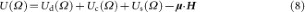where Ud(Ω), Uc(Ω), Us(Ω) and − μ·H are the shape, crystalline and surface anisotropy energies and Zeeman energy, respectively and Ω is the solid angle of μ . For spheroidal particles, the first term, Ud(Ω), can be described as KdVsin2 φ and has two minima separated by the energy barrier ΔU = KdV, where Kd is the shape anisotropy constant and φ is the angle between the long (easy) axis and μ . The magnitudes of Kd, given by (NeasyNhard0·Ms2, are 5 and 16 kJ/m3 for spheroidal particles with κ of 1.1 and 1.4, respectively. Neasy and Nhard are the demagnetising factors for the long and short axes, respectively. In comparison, magnetite has cubic crystalline anisotropy20 and an anisotropy constant K1 = −11 kJ/m3. The energy landscape of cubic anisotropy (K1<0) is gentle and ΔU is (1/12)K1V ≈ (1 kJ/m3V19. Additionally, the effects of surface anisotropy are generally insignificant if the particles are larger than 10 nm18, although the rationale for this is still unknown. For these reasons, we assumed that the uniaxial shape anisotropy dominated. Consequently, equation (8) can be simplified as follows: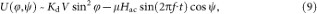where ψ is the angle between μ and H (see Fig. 3).

Little is known about the local environments of magnetic nanoparticles accumulated in tumour tissue. For example, nanoparticles coated with dextran are completely immobilised in tissues21, whereas dextran nanoparticles appear to be mobile in cells5. For this reason, we simulated the magnetic response of the monodisperse spheroidal nanoparticles in the following two extreme cases: non-rotatable nanoparticles with randomly oriented easy axes and rotatable nanoparticles in a Newtonian fluid with a viscosity η of 1 mPa·s.4

### Simulation of the reversal

The detailed trajectories of μ in a magnetic field applied at an oblique angle θ to the long easy axis of a spheroidal particle can be precisely simulated by solving the stochastic Landau–Lifshitz–Gilbert equations. However, we are only interested in the reversal of μ once every microsecond because the frequency used for hyperthermia is limited. Therefore, we can use a well-known coarse-grained approach, or “two-level approximation”14, that considers thermally activated reversals between the meta-stable directions, (φ 1, ψ 1) and (φ 2, ψ 2), via the midway saddle point at (φ 3, ψ 3) in Uθ(φ,ψ). The reversal probability from (φ 1, ψ 1) to (φ 2, ψ 2), υ 12, is given by f0–1·exp[(Uθ3, ψ 3) − Uθ1, ψ 1))/kBT ], while the backward reversal probability υ 21 is f0–1·exp[(Uθ3, ψ 3) − Uθ2, ψ 2))/kBT ], where f0 is the attempt frequency of 109 s–1.

In the simulation, the time evolution of the occupation probabilities, p1(θ), p2(θ) = 1 − p1(θ), at the two stable directions of a nanoparticle tilted at θ was computed using the following relationship: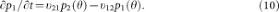p1(θ) was simply set to either zero or one when there was only one minimum in Uθ(φ,ψ). This calculation was continued until the transient factors depending on the initial conditions disappeared. The time step Δt was typically 10−4/f s but was shorter when υ 12Δt (or υ 21Δt) became large compared with one. At each step, magnetisation was obtained as ∫(p1(θ)μcosψ 1 + p2(θ)μcosψ 2)sinθdθ. Test simulations were performed to check the validity of this method using the same parameters as in an earlier study14 to calculate the reversals of magnetic nanoparticles in large AC magnetic fields. As detailed in the Supplementary Information, our results agree with the reported behaviour14.

### Simulation of reversal and rotation

The rotation of spheroidal nanoparticles was simulated in synchronisation with the reversal of μ . In Newtonian fluids, the frictional torque for rotation can be expressed as 6ηVH·(0.8 + 0.2κ)· ω (t),22 where VH = [(4/3)π·κ(RM + δR)3] is the hydrodynamic volume and ω (t) is the angular velocity of rotation; d e /dt = ω (t) × e (t), where e (t) is the unit vector along the long axis of the spheroid; and μ (te (t) = μcosφ. Under typical conditions, where η = 1 mPa·s, VH ≈ 103 nm3 and ω(t) ≈ 1×105 rad/s, the inertia of the nanoparticle can be neglected (Brownian dynamics simulation). In this inertia-less limit23, the frictional torque balances with magnetic torque μ (tH (t) and Brownian torque λ (t) as follows: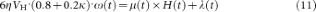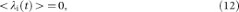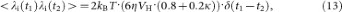where δ(t1−t2) is the Dirac delta function.

At the beginning of the simulation for the rotatable nanoparticles, an assembly of randomly oriented nanoparticles was generated, where their number ensures an optimal compromise between calculation time and precision. Then, the time evolution of the direction of μ and the orientation of the long easy axis were computed by the following steps. (i) Using equation (9), reversible variations of the meta-stable directions (φ i(t), ψ i(t)) caused by the latest changes in the field strength and direction of the easy axis were calculated, (ii) μ (t) at (φ 1(t), ψ 1(t)) was reversed if x < υ 12Δt, but otherwise not. In this case, x [0, 1] is a pseudorandom number generated by the Xorshift algorithm24. The backward reversal was computed in a similar manner. (iii) Substituting the reversed (or held) μ (t) into equation (11), ω (t) was calculated. (iv) e (t) was finally computed using the relationship d e /dt = ω (te (t). This calculation was continued until transient factors depending on the initial conditions disappeared. In this simulation, Δt was typically 10−4/f s but was shorter unless υ 12Δt, or the changes in e (t) were sufficiently small compared to one. Magnetisation was obtained as Σ(ni μcosψ i) at each step. Test simulations were performed to check the validity of this method. There have been no prior theoretical studies on systems where both reversal and rotation occur simultaneously in a large AC magnetic field. Consequently, comparisons with prior studies were performed under two extreme conditions. In the first case, high viscosities were assumed. Because reversal dominates rotation under these conditions, the results were compared with those reported by Carrey et al.14. In the second case, a high anisotropic field was assumed. Because rotation dominates reversal in this situation, the results were compared with the numerical simulations of Yoshida et al., where nonlinear Brownian rotational relaxation of magnetic fluids with a large excitation field was studied using the Fokker–Planck equation13. The results obtained from our simulation of reversal and rotation were consistent with those of earlier studies (Supplementary Figs. S1–S4). Therefore, we can now take a first step toward understanding the roles that rotation of a nanoparticle and reversal of its magnetic moment play together in large AC magnetic fields.

## References

• Pankhurst, Q. A., Thanh, N. K. T., Jones, S. K. & Dobson, J. Progress in applications of magnetic nanoparticles in biomedicine. J. Phys. D42 224001 (2009).

• Sugahara, K. N., et al. Coadministration of a tumor-penetrating peptide enhances the efficacy of cancer drugs. Science 328, 1031–1035 (2010).

• DeNardo, S. J., et al. Development of Tumor Targeting Bioprobes (111In-Chimeric L6 Monoclonal Antibody Nanoparticles) for alternating magnetic field cancer therapy. Clin. Cancer Res. 11, 7087s–7092s (2005).

• Kuimova, M. K., et al. Imaging intracellular viscosity of a single cell during photoinduced cell death. Nature Chem. 1, 69−73 (2009).

• Kalwarczyk, T., et al. Comparative analysis of viscosity of complex liquids and cytoplasm of mammalian cells at the nanoscale. Nano Lett. 11, 2157–2163 (2011).

• Rosensweig, R. E. Heating magnetic fluid with alternating magnetic field. J. Magn. Magn. Mater. 252, 370–374 (2002).

• Chung, S. -H., et al. Biological sensing with magnetic nanoparticles using Brownian relaxation. J. Appl. Phys. 97, 10R101 (2005).

• Kalele, S., Narain, R. & Krishnan, K. M. Probing temperature-sensitive behaviour of pNIPAAm-coated iron oxide nanoparticles using frequency-dependent magnetic measurements. J. Magn. Magn. Mater. 321, 1377–1380 (2009).

• Hergt, R., Dutz, S. & Röder, M. Effects of size distribution on hysteresis losses of magnetic nanoparticles for hyperthermia. J. Phys.: Condens. Matter 20, 385214 (2008).

• Kim, D.-H., Nikles, D. E., Johnson, D. T. & Brazel, C. S. Heat generation of aqueously dispersed CoFe2O4 nanoparticles as heating agents for magnetically activated drug delivery and hyperthermia. J. Magn. Magn. Mater. 320, 2390–2396 (2008).

• Müller, R., et al. Hysteresis losses in iron oxide nanoparticles prepared by glass crystallization or wet chemical precipitation. J. Magn. Magn. Mater. 310, 2399–2404 (2007).

• Brown, Jr, W. F. Thermal Fluctuations of a Single-Domain Particle. Phys. Rev. 130, 1677–1686 (1963).

• Yoshida, T., & Enpuku, K. Simulation and Quantitative Clarification of AC Susceptibility of Magnetic Fluid in Nonlinear Brownian Relaxation Region, Jpn . J. Appl. Phys. 48, 127002 (2009).

• Carrey, J., Mehdaoui, B., & Respaud, M. Simple models for dynamic hysteresis loop calculations of magnetic single-domain nanoparticles: Application to magnetic hyperthermia optimization, J. Appl . Phys. 109, 083921 (2011).

• Fortin, J. P., et al. Size-sorted anionic iron oxide nanomagnets as colloidal mediators for magnetic hyperthermia, J. Am . Chem. Soc. 129, 2628−2635 (2007).

• Mamiya, H., Nakatani, I., & Furubayashi, T. Phase transitions of iron nitride magnetic fluids, Phys . Rev. Lett. 84, 6106−6109 (2000).

• Li, Z., Kawashita, M., Araki, N., Mitsumori, M., Hiraoka, M. & Doi, M. Magnetite nanoparticles with high heating efficiencies for application in the hyperthermia of cancer. Mater. Sci. Engineering, C 30, 990–996 (2010).

• Demortière, P., et al. Size-dependent properties of magnetic iron oxide nanocrystals. Nanoscale 3, 225−232 (2011).

• Mamiya, H., & Jeyadevan, B. Optimal design of nanomagnets for targeted hyperthermia J. Magn . Magn. Mater. 323, 1417–1422 (2011).

• Hellwege, K. H. Magnetic and Other Properties of Oxides and Related Compounds, (Landolt-Börnstein New Series III 4b, Springer-Verlag, New York, 1970).

• Dutz, S., Kettering, M., Hilger, I., Müller, R., & Zeisberger, M. Magnetic multicore nanoparticles for hyperthermia—influence of particle immobilization in tumour tissue on magnetic properties,. Nanotechnology 22, 265102 (2011)

• Ilg, P. & Kröger, M. Magnetisation dynamics, rheology and an effective description of ferromagnetic units in dilute suspension. Phys. Rev. E66, 021501 (2002).

• Coffey, W. T. On the contribution of multiplicative noise terms to the Langevin equation for rotational relaxation. J. Chem. Phys. 99, 3014–3020 (1993).

• Marsaglia, G. Xorshift RNGs, J. Stat . Soft. 8, 1–6 (2003).

## Acknowledgements

This study was partly supported by a Grant-in-Aid for Scientific Research (21241023 and 21681014).

## Author information

Authors

### Contributions

H. M. performed the simulation. H. M. and B. J. wrote the manuscript.

## Ethics declarations

### Competing interests

The authors declare no competing financial interests.

## Electronic supplementary material

### Supplementary Information

Supplementary materials

## Rights and permissions

Reprints and Permissions

Mamiya, H., Jeyadevan, B. Hyperthermic effects of dissipative structures of magnetic nanoparticles in large alternating magnetic fields. Sci Rep 1, 157 (2011). https://doi.org/10.1038/srep00157

• Accepted:

• Published:

• DOI: https://doi.org/10.1038/srep00157

• ### Properties of assembly of superparamagnetic nanoparticles in viscous liquid

• N. A. Usov
• R. A. Rytov
• V. A. Bautin

Scientific Reports (2021)

• ### Transmittance Measurements in Non-alternating Magnetic Field as Reliable Method for Determining of Heating Properties of Phosphate and Phosphonate Coated Fe3O4 Magnetic Nanoparticles

• Marija Mirković
• Marko Perić

Journal of Inorganic and Organometallic Polymers and Materials (2021)

• ### Heating Efficiency of Triple Vortex State Cylindrical Magnetic Nanoparticles

• De Wei Wong
• Wei Liang Gan
• Wen Siang Lew

Nanoscale Research Letters (2019)

• ### The relevance of Brownian relaxation as power absorption mechanism in Magnetic Hyperthermia

• Teobaldo E. Torres
• Enio Lima
• Gerardo F. Goya

Scientific Reports (2019)

• ### Influence of Viscosity on Dynamic Magnetization of Thermally Blocked Iron Oxide Nanoparticles Characterized by a Sensitive AC Magnetometer

• Mohd Mawardi Saari
• Nazatul Shareena Suhaimi

Journal of Superconductivity and Novel Magnetism (2019)